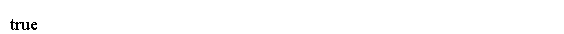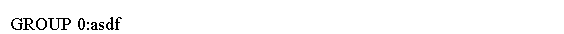# Java 正则表达式元字符

2023-02-09 15:14 更新

## Java正则表达式教程 - Java正则表达式元字符

Java中的正则表达式支持的元字符如下:

`( ) [ ] { } \ ^ \$ | ? * + . < > - = !`

## 字符类

` ^ `表示不是。

` [a-zA-Z0-9] `匹配任何字符a到z(大写和小写)和任何数字0到9。

[abc] 字符a，b或c
[^xyz] 除x，y和z以外的字符
[a-z]
[a-cx-z] 字符a到c或x到z，其将包括a，b，c，x，y或z。
[0-9&&[4-8]] 两个范围(4,5,6,7或8)的交叉，
[a-z&&[^aeiou]] 所有小写字母减元音

## 预定义字符类

含义
. 任何字符
\d 数字。 与[0-9]相同
\D 非数字。 与[^ 0-9]相同
\s 空格字符。 包括与[\\ t \\ n \\ x0B \\ f \\ r]相同。
• 空格
• 标签
• 换行符
• 垂直标签
• 表单Feed
• 回车字符
\S 非空白字符。 与[^ \\ s]相同
\w 一个字符。 与[a-zA-Z_0-9]相同。
\W 非字字符。 与[^ \ w]相同。

## 例子

` \\d `在字符串中用于转义` \ `

```import java.util.regex.Matcher;
import java.util.regex.Pattern;

public class Main {
public static void main(String args[]) {
Pattern p = Pattern.compile("Java \\d");
String candidate = "Java 4";
Matcher m = p.matcher(candidate);

if (m != null)
System.out.println(m.find());
}
}```## 例2

```import java.util.regex.Matcher;
import java.util.regex.Pattern;

public class Main {
public static void main(String args[]) {
String regex = "\\w+";
Pattern pattern = Pattern.compile(regex);

String candidate = "asdf Java2s.com";
Matcher matcher = pattern.matcher(candidate);
if (matcher.find()) {
System.out.println("GROUP 0:" + matcher.group(0));
}
}
}```App下载